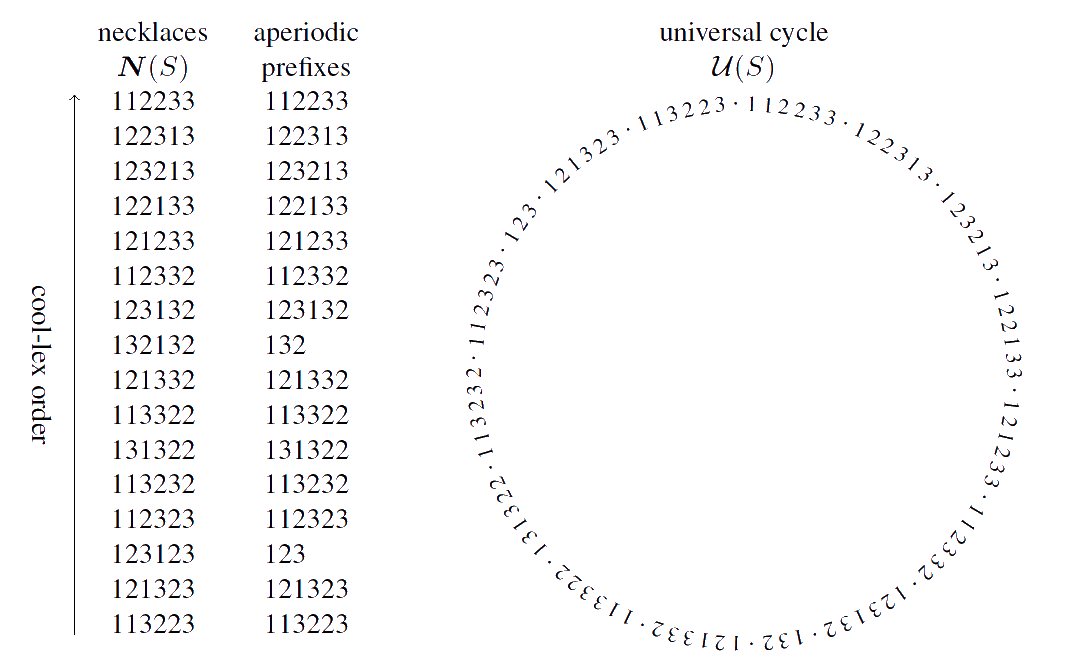#De Bruijn Sequence and Universal Cycle Constructions

Cool-lex Concatenation Output
 Symbols $k$ 2 3 4 5 6 7 8 9 Content ($n_1~n_2 \cdots n_k$)
Constructing universal cycles for strings with fixed-content (multiset permutations)

Permutations and fixed-weight (where the weight is the number of 1s) binary strings are special cases of strings with fixed-content, or multiset permutations. For permutations, the content is $\{1,2,\ldots, n\}$ and for binary strings with fixed-weight $w$ the content consists of $w$ 1s and $n-w$ 0s. For each of these special cases, cool-lex order has been applied to construct universal cycles for the shorthand representations of these sets [HRW12,RSW12]. Recently, these results have been generalized for fixed-content strings [SW21], again using a shorthand representation (ignoring the final redundant symbol of each string).

A necklace is the lexicographically smallest string in an equivalence class under rotation. The aperiodic prefix of a necklace $\alpha$ is the shortest string $\beta$ such that $\beta^j = \alpha$ for some integer $j$. The following is a remarkably simple, and somewhat amazing algorithm to construct a universal cycle for fixed-content strings using their shorthand representation.

Necklace Concatenation Algorithm with Cool-lex Order

Concatenate together the aperiodic prefixes of the necklaces with fixed-content as they appear in reverse cool-lex order.

There are iterative and recursive algorithms for generating necklaces with fixed-content in cool-lex order given in [SW21]. Applying the recursive algorithm, the above universal cycle can be constructed in $O(1)$-amortized time per symbol.

Example for content $S=\{1,1,2,2,3,3\}$

Observe that all 90 shorthand strings (of length 5) for original content $S$ appear exactly once in the universal cycle below.The UC generated from this concatenation construction has a successor-rule based on the Missing Symbol Resister (MSR) that can be derived by considering the first inversion in the underlying cycles [SW22].

Cool-lex UC-successor

$f(\sigma) = \left\{ \begin{array}{ll} p_1 & \ \ \mbox{if$z > p_1$and$h(z p_1 p_2 \cdots p_{n-1})$is a necklace and$z \geq p_{n-1}$;} \\ p_1 & \ \ \mbox{if$p_1 > z$and$h(p_1 z p_2 \cdots p_{n-1})$is a necklace and$b_1 \geq p_{n-1}$;} \\ z \ &\ \ \mbox{otherwise,}\end{array} \right.$

where $z$ is the missing symbol in the shorthand multiset permutation $\sigma = p_1p_2\cdots p_{n-1}$ and $h(\pi)$ is the rotation of the multiset permutation $\pi = q_1q_2\cdots q_n$ that takes the longest non-decreasing suffix of $q_3\cdots q_n$ and rotates it to the front of $\pi$. For example $h(12332\color{blue}{1233}) = \color{blue}{1233}12332$.